# NCERT Solutions for Class 7 Maths Chapter 11 Perimeter and Area Ex 11.2

NCERT Solutions for Class 7 Maths Chapter 11 Perimeter and Area Ex 11.2

### NCERT Solutions for Class 7 Maths Chapter 11 Perimeter and Area Ex 11.2

NCERT Solutions for Class 7 Maths Chapter 11 Perimeter and Area Exercise 11.2
Ex 11.2 Class 7 Maths Question 1.
Find the area of each of the following parallelograms: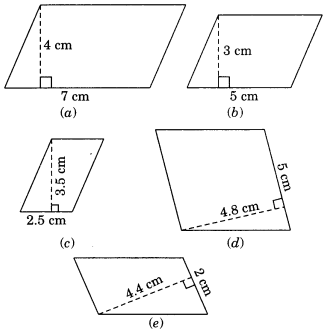Solution:
(a) Area of the parallelogram
= base × altitude = 7 cm × 4 cm
= 28 cm2
(b) Area of the parallelogram
= base × altitude = 5 cm × 3 cm
= 15 cm2
(c) Area of the parallelogram
= base × altitude = 2.5 cm × 3.5 cm
= 8.75 cm2
(d) Area of the parallelogram
= base × altitude = 5 cm × 4.8 cm
= 24.0 cm2
(e) Area of the parallelogram
= base × altitude = 2 cm × 4.4 cm
= 8.8 cm2

Ex 11.2 Class 7 Maths Question 2.
Find the area of each of the following triangles: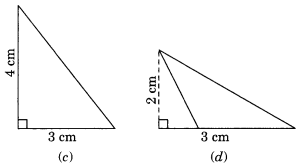Solution:
Area of the triangle = 1/2 × b × h
1/2 × 4 cm × 3 cm
= 6m2

(b) Area of the triangle = 1/2 × b × h
1/2 × 5 cm × 3.2 cm
= 8.0 cm2

(c) Area of the triangle = 1/2 × b × l
1/2 × 3 cm × 4 cm
= 6 cm2

(d) Area of the triangle = 1/2 × b × h
1/2 × 3 cm × 2 cm
= 3 cm2

Ex 11.2 Class 7 Maths Question 3.
Find the missing values:

 S.No. Base Height Area of the parallelogram (a) 20 cm 246 cm2 (6) 15 cm 154.5 cm2 (c) 8.4 cm 48.72 cm2 (d) 15.6 16.38 cm2

Solution:
(a) Area of the parallelogram =b × h
246 = 20 × h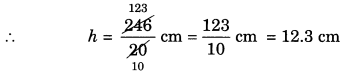(b) Area of the parallelogram = b × h
154.5 = b × 15(c) Area of the parallelogram = b × h
48.72 = b × 8.4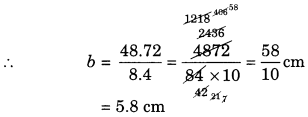(d) Area of the parallelogram = b × h
16.38 = 15.6 × h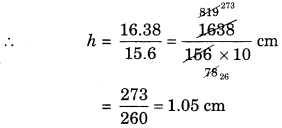Ex 11.2 Class 7 Maths Question 4.
Find the missing values:

 Base Height Area of the triangle 15 cm — 87 cm2 — 31.4 mm 1256 mm2 22 cm — 170.5 cm2

Solution:
(i) Area of the triangle = 1/2 × b × hSo, the height =11.6 cm

(ii) Area of the triangle = 1/2 × b × hSo, the required base = 80 mm.

(iii) Area of the triangle = 1/2 × b × h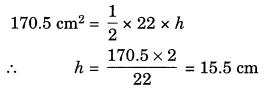So, the required height = 15.5 cm

Ex 11.2 Class 7 Maths Question 5.
PQRS is a parallelogram. QM is the height of Q to SR and QN is the height from Q to PS. If SR = 12 cm and QM = 7.6 cm. Find:
(a) the area of the parallelogram PQRS
(b) QN, if PS = 8 cm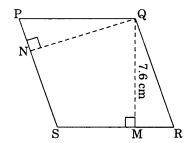Solution:
(a) Area of the parallelogram PQRS
= SR × QM (∵ Area = Base × Height)
= 12 cm × 7.6 cm
= 91.2 cm2

(b) Area of the parallelogram PQRSEx 11.2 Class 7 Maths Question 6.
DL and BM are the heights on sides AB and AD respectively of parallelogram ABCD. If the area of the parallelogram is 1470 cm2, AB = 35 cm and AD = 49 cm, find the length of BM and DL.Solution:
Area of the parallelogram ABCD
= AB × DL
⇒ 1470 cm2 = 35 cm × DL
⇒ 1470/35 DL
∴ DL = 42 cm
Area of the parallelogram ABCD = AD × BM
1470 cm2 = 49 cm × BM
⇒ 1470/49 = 30 cm
∴ BM = 30 cm
Hence, BM = 30 cm and DL = 42 cm

Ex 11.2 Class 7 Maths Question 7.
∆ABC is right angled at A. AD is perpendicular to BC. If AB = 5 cm, BC = 13 cm and AC = 12 cm, find the area of ∆ABC. Also find the length of AD.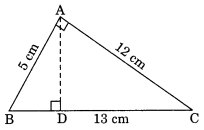Solution:
Area of right triangle ABC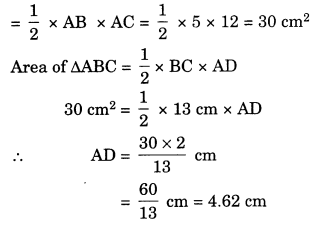Ex 11.2 Class 7 Maths Question 8.
∆ABC is isosceles with AB = AC = 7.5 cm and BC = 9 cm. The height AD from A to BC, is 6 cm. Find the area of ∆ABC. What will be the height from C to AB i.e., CE?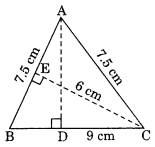Solution:
Area of ∆ABC = 1/2 × base × height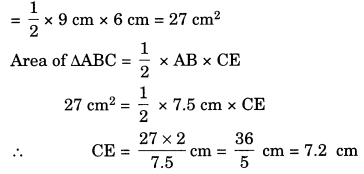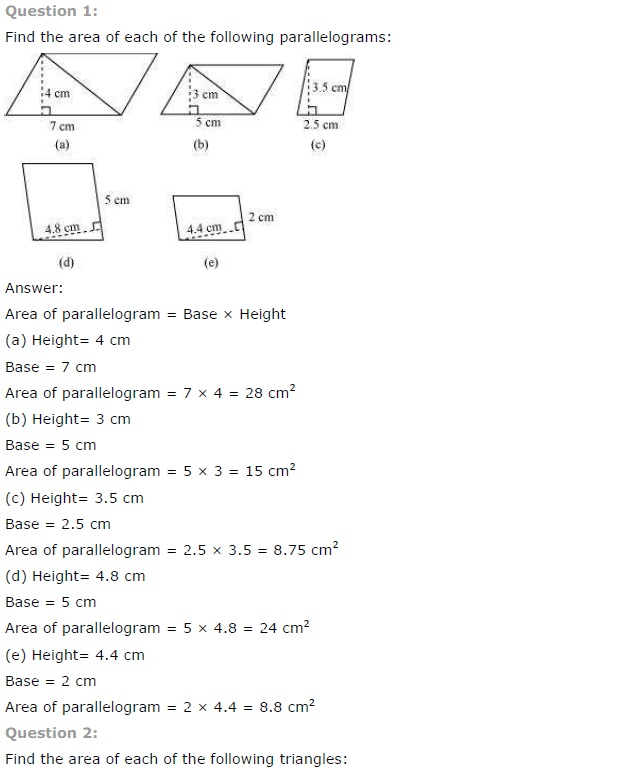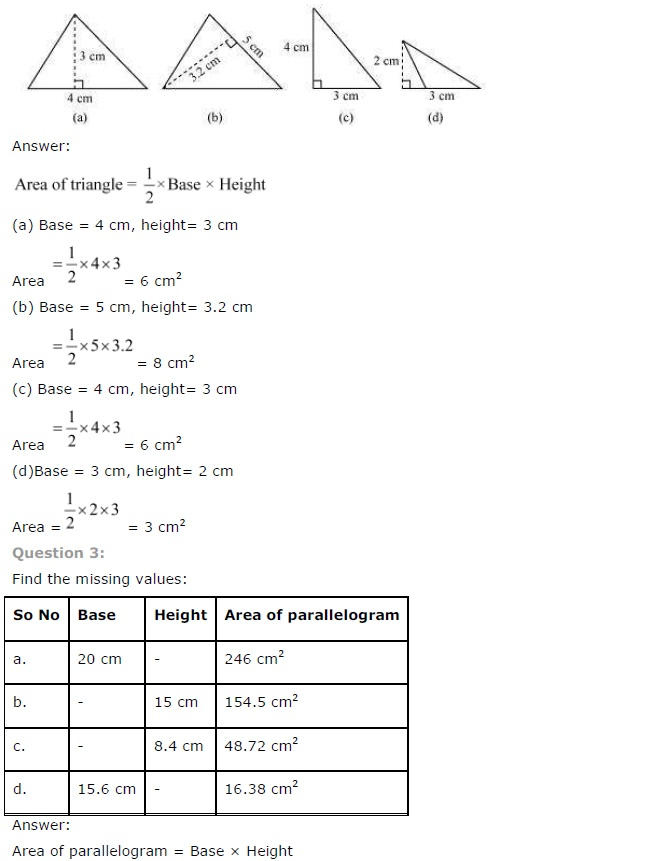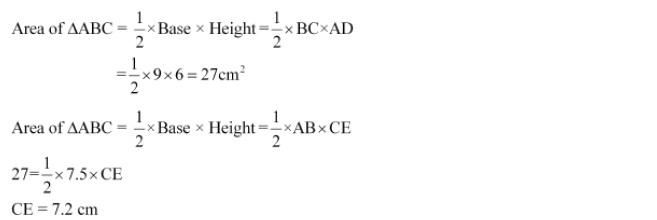## SabDekho

The Complete Educational Website